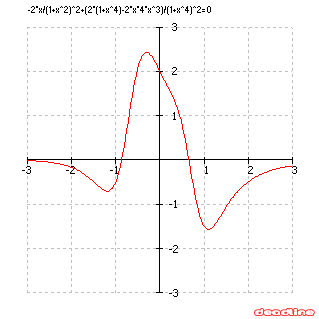﻿ Solve exponential equations: -2*x/(1+x^2)^2+(2*(1+x^4)-2*x*4*x^3)/(1+x^4)^2 = 0

# Solve exponential equations

Problem: solve an exponential equation

Equation to solve: -2*x/(1+x^2)^2+(2*(1+x^4)-2*x*4*x^3)/(1+x^4)^2 = 0

Interval for roots: [-3, 3]

DeadLine can solve exponential equations instantly, providing the equation graph and the roots.

Number of roots found: 2

 x1 -0,86650135 x2 0,6545409